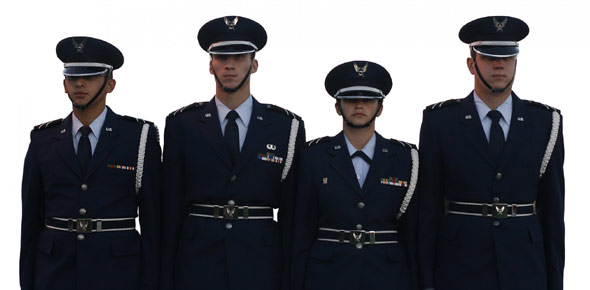# 2A672 Volume 1 Unit Review

7 Questions | Total Attempts: 145SettingsAerospace Ground Support Mechanic 7 level CDC

• 1.
What is the simplest form of matter
• A.

Element

• B.

Mixture

• C.

Electrons

• D.

Compound

• 2.
In an atom, what electrons contain the most energy?
• A.

The energy is shared equally by all electrons

• B.

Orbiting electrons

• C.

Valence electrons

• D.

K shell electrons

• 3.
Atoms havign more than four electrons, but less than eight are known as
• A.

Insulators

• B.

Conductors

• C.

Stable atoms

• D.

Semiconductors

• 4.
The electrostatic fields around a positive ion move
• A.

Inward

• B.

Outward

• C.

Inward then outward

• D.

Outward then inward

• 5.
What are factors that affect the resistence of a material
• A.

Area, weight , and state of material

• B.

Area, Temperature, and type of material

• C.

Temperature, weight, and state of material

• D.

Temperature,, type of material, and state of material

• 6.
Wlhat is the resistence in a circuit if the power rating is 40watts and the total current is 2 amps
• A.

40 Ohms

• B.

20 Ohms

• C.

10 Ohms

• D.

0.5 Ohms

• 7.
Convert 500 milliamps to standard amperage
• A.

.005 amps

• B.

.05 amps

• C.

.5 amps

• D.

50 amps

Related TopicsBack to top